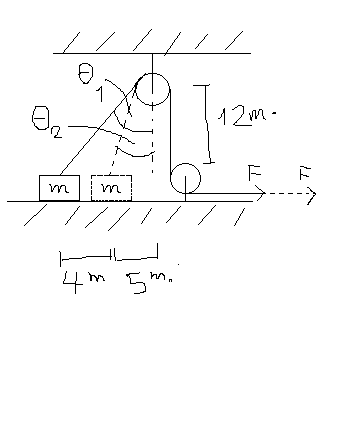# No calculus required!From the figure (sorry for "paint" quality XD). A mass $m$ is placed on a frictionless surface with angle $\theta_{1}$ to the vertical line. It's pulled by constant force $F = 42.25 \text{ newton}$ using a massless string such that the mass don't float when it's pulled. After I stop pulling this mass when the rope does the angle $\theta_{2}$ to the vertical line, find the amount of work done by $F$ in $\text{joules}$ at the point when I stop pulling it.

Details:

• Pulleys are very strong that they always stay still, massless, and frictionless.
• $g = 9.8 \frac{m}{s^{2}}$
• $m = 25.1 kg$
• This problem is from my physics teacher.
×

Problem Loading...

Note Loading...

Set Loading...